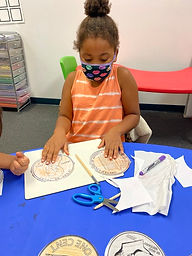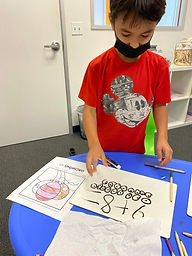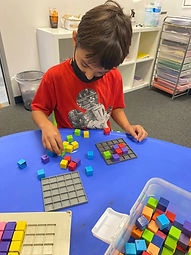## Ms. Caitlyn

### Target 1​

###### Lesson Type:

New

Number Operation

:

Fractional Numbers

Identify US currency coins.

###### 1:

Visually distinguish between a dime and penny.

###### 2:

Become familiar with both the front and back sides of dimes and pennies.

###### 3:

Know the value of dimes and pennies.

1st

###### Vocabulary:

Dime, Penny, Cents, Value

Activities:

Students observed dimes and pennies and compared/contrasted their characteristics. They were able to touch, play with, and look at each of the coins to observe and get a feel of differences in the coins.

Students created their own enlarged dimes and pennies in order to understand the characteristics (size, color, features of the front/back).

Students used dice to make different amounts of money using dimes and pennies. This can be a segway into adding within 50, as they have to add the coin values together.### Home Exploration

###### Guiding Questions:## Absent Students:

### Target 2

:

###### 1:

Recognize the arithmetic symbols in an addition equation: plus (+) and equals (=).

###### 2:

Understand that addition means to put two quantities together.

###### 3:

Understand that adding numbers means making a larger number.

###### 4:

Count on from a given number to add another quantity.

###### 5:

Understand that addition equations can be written both vertically and horizontally.

1st

###### Vocabulary:

Add, Plus, Equals To, Vertical, Horizontal

Activities:

Students rolled dice/spun the spinner to generate addition problems for practice. They counted manipulatives of choice in order to solve.

Students completed an addition color-by-number worksheet. They used manipulatives, counting on their fingers, and drawing on their whiteboards to complete the tasks.### Home Exploration

###### Guiding Questions:### Target 3

:

###### 1:

Use the given information in a problem to identify the correct answer.

###### 2:

Use the given information to determine which is not the correct answer.

###### 3:

Think outside of the box to solve problems.

###### 4:

Follow given rules to solve problems.

2nd

###### Vocabulary:

Color, Space, Visualize, Sudoku

Activities:

Students played color sudoku using array boards.### Home Exploration